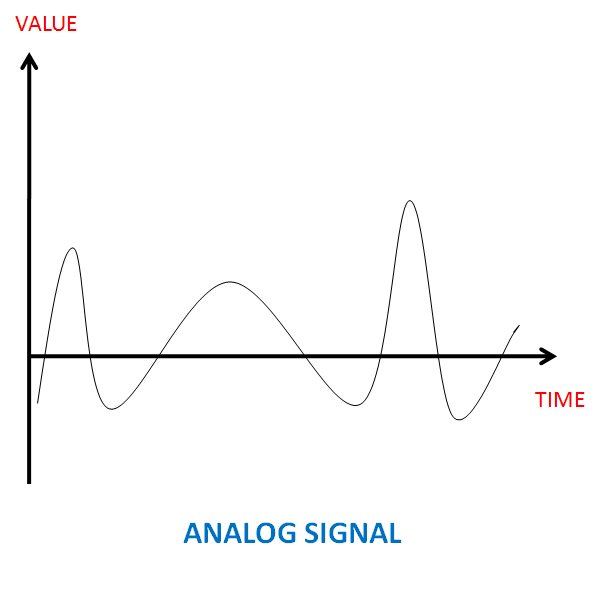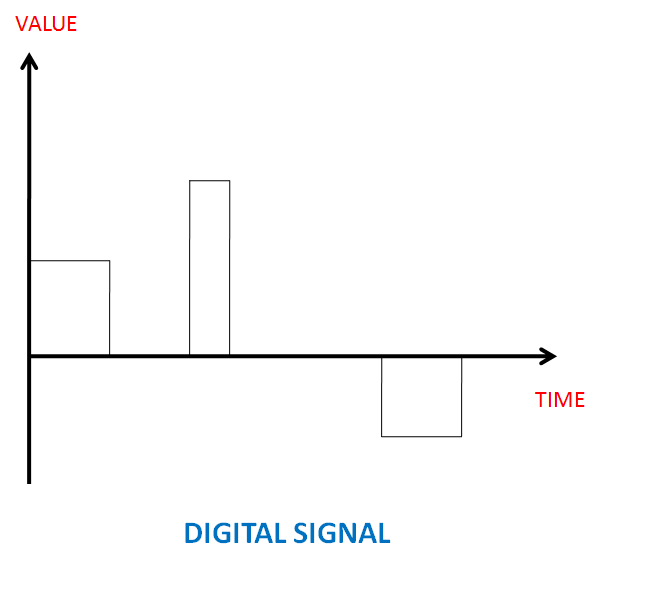Looking for NEET / JEE Coaching? Enquire Now

# Physical Layer of internet model: Analog, Digital Data and Signal

This introduces us to the physical layer of the internet model. You will learn about the tasks undertaken by this layer and the transmission requirements for it. You will also be introduced to the basics of analog and digital transmission.

In this article we came across the uses of computer network. Certain layered models are followed that help in data transmission and here we would explore one such layer. Physical layer is the bottom-most layer of the Internet Model and is responsible for the interaction with the transmission media i.e. the physical part of the network which connects the components together. As a result of this the layer is responsible for carrying information from one node to the other.

Of the many tasks that are performed by the physical layer, one is to provide service to the data link layer. This layer consists of 0s and 1s which are organized into frames and need to be transmitted across the transmission medium. Thus the physical layer has to convert these binary values into signals so that it can be transmitted. This layer also needs to decide the total number of logical channels required for transmitting the data. It is so because the transmission medium in itself is passive and can't decide the flow direction on its own.

## Analog and Digital Signal

Data or the signal whichever is used in a network, it can be either digital or analog.

### Analog and Digital Data

Analog data refers to data that is of continuous format whereas digital data is one which has discrete states. So the analog data takes continuous values and digital data takes discrete values. Analog data can be directly converted into an analog signal or sampled and converted to digital signal. In quite a similar fashion digital data can also be converted to digital signal or into analog signal after modulation. These are converted so that efficient transmission can take place.

### Analog and Digital Signal

Similar to data, the signals which represent these can also be digital or analog. Analog signals are known to have many levels of intensity over a given period of time. As the wave moves from one value to another, along the path it traverses via infinite number of values. Digital signals rather have only definite set of values. These are represented using a pair of perpendicular axes. The vertical axis represents the strength of the signal and the horizontal axis gives the time period.As visible from the above images that for analog signal the values are continuous since it passes through infinite number of points. Digital signal demonstrates sudden jumps this is due to the finite values and the transition from one value to another.

### Periodic and Non-Periodic Signals

Periodic signal is one which completes a pattern within a given measurable time frame. This particular time frame is called a period. Cycle refers to the completion of one complete pattern. On the other hand a non-periodic or aperiodic signal does not exhibit any pattern or cycle over a given time period. Analog and digital signals can be either periodic or non-periodic.

Usually analog signals are preferred since they require lesser bandwidth. Furthermore non-periodic signals are given preference as they efficiently represent variation in data. Thus the generally used combination arises to be periodic analog signals and non-periodic digital signals.